### Practice Set 5.4 Probability Class 10th Mathematics Part 1 MHB Solution

Practice Set 5.4
1. If two coins are tossed, find the probability of the following events. (1) Getting at…
2. If two dice are rolled simultaneously, find the probability of the following events.…
3. There are 15 tickets in a box, each bearing one of the numbers from 1 to 15. One ticket…
4. A two digit number is formed with digits 2, 3, 5, 7, 9 without repetition. What is the…
5. A card is drawn at random from a pack of well shuffled 52 playing cards. Find…
###### Practice Set 5.4
Question 1.

If two coins are tossed, find the probability of the following events.

(1) Getting at least one head.

Sample Space, S=(HH,HT,TH,TT)

(1)Probability of getting at least one head, p(A)=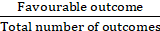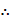p(A)=(3) Probability of getting no head, p(B)=p(B)=Question 2.

If two dice are rolled simultaneously, find the probability of the following events.

(1) The sum of the digits on the upper faces is at least 10.

(2) The sum of the digits on the upper faces is 33.

(3) The digit on the first die is greater than the digit on second die.

(1) Probability of getting the sum of the digits on the upper faces is at least 10, p(A)=We know the Favourable Cases are (Where the Sum of digits on the upper faces is at least 10):- (4,6), (5,5), (5,6), (6,4), (6,5), (6,6)- 6 cases

Total Number of Outcomes:- 36p(A)=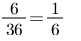(2) Probability of getting the sum of the digits on the upper faces is 33, p(B)=Favorable Outcomes(Getting the sum of Digits on the upper Faces is 33)= 0 as the maximum sum could be 12p(B)=0

(3) (3) Probability of getting the digit on the first die is greater than the digit on second die, p(C)=Favorable Outcomes are as follow:- (2,1), (3,1), (3,2), (4,1), (4,2), (4,3), (5,1), (5,2), (5,3), (5,4), (6,1), (6,2), (6,3),(6,4), (6,5)= 15 casesp(C)=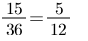Question 3.

There are 15 tickets in a box, each bearing one of the numbers from 1 to 15. One ticket is drawn at random from the box. Find the probability of event that the ticket drawn -

(1) shows an even number.

(2) shows a number which is a multiple of 5.

(1) Probability of event that the ticket drawn shows an even number, p(E) =p(E)=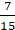(2) Probability of event that the ticket drawn shows a number which is a multiple of 5,

p(X) =p(X)=Question 4.

A two digit number is formed with digits 2, 3, 5, 7, 9 without repetition. What is the probability that the number formed is

(1) an odd number ?

(2) a multiple of 5 ?

(1) Probability that the number formed is an odd number,

p(X) =p(X)=(2) Probability that the number formed is an odd number,

p(X) =p(X)=Question 5.

A card is drawn at random from a pack of well shuffled 52 playing cards. Find the probability that the card drawn is -

(1) an ace. (2) a spade.

(1) Probability that the card drawn is an ace, p(A)=p(A)=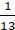(2) Probability that the card drawn is a spade, p(B)=p(B)=## PDF FILE TO YOUR EMAIL IMMEDIATELY PURCHASE NOTES & PAPER SOLUTION. @ Rs. 50/- each (GST extra)

SUBJECTS

HINDI ENTIRE PAPER SOLUTION

MARATHI PAPER SOLUTION
SSC MATHS I PAPER SOLUTION
SSC MATHS II PAPER SOLUTION
SSC SCIENCE I PAPER SOLUTION
SSC SCIENCE II PAPER SOLUTION
SSC ENGLISH PAPER SOLUTION
SSC & HSC ENGLISH WRITING SKILL
HSC ACCOUNTS NOTES
HSC OCM NOTES
HSC ECONOMICS NOTES
HSC SECRETARIAL PRACTICE NOTES

2019 Board Paper Solution

HSC ENGLISH SET A 2019 21st February, 2019

HSC ENGLISH SET B 2019 21st February, 2019

HSC ENGLISH SET C 2019 21st February, 2019

HSC ENGLISH SET D 2019 21st February, 2019

SECRETARIAL PRACTICE (S.P) 2019 25th February, 2019

HSC XII PHYSICS 2019 25th February, 2019

CHEMISTRY XII HSC SOLUTION 27th, February, 2019

OCM PAPER SOLUTION 2019 27th, February, 2019

HSC MATHS PAPER SOLUTION COMMERCE, 2nd March, 2019

HSC MATHS PAPER SOLUTION SCIENCE 2nd, March, 2019

SSC ENGLISH STD 10 5TH MARCH, 2019.

HSC XII ACCOUNTS 2019 6th March, 2019

HSC XII BIOLOGY 2019 6TH March, 2019

HSC XII ECONOMICS 9Th March 2019

SSC Maths I March 2019 Solution 10th Standard11th, March, 2019

SSC MATHS II MARCH 2019 SOLUTION 10TH STD.13th March, 2019

SSC SCIENCE I MARCH 2019 SOLUTION 10TH STD. 15th March, 2019.

SSC SCIENCE II MARCH 2019 SOLUTION 10TH STD. 18th March, 2019.

SSC SOCIAL SCIENCE I MARCH 2019 SOLUTION20th March, 2019

SSC SOCIAL SCIENCE II MARCH 2019 SOLUTION, 22nd March, 2019

XII CBSE - BOARD - MARCH - 2019 ENGLISH - QP + SOLUTIONS, 2nd March, 2019

HSC Maharashtra Board Papers 2020

(Std 12th English Medium)

HSC ECONOMICS MARCH 2020

HSC OCM MARCH 2020

HSC ACCOUNTS MARCH 2020

HSC S.P. MARCH 2020

HSC ENGLISH MARCH 2020

HSC HINDI MARCH 2020

HSC MARATHI MARCH 2020

HSC MATHS MARCH 2020

SSC Maharashtra Board Papers 2020

(Std 10th English Medium)

English MARCH 2020

HindI MARCH 2020

Hindi (Composite) MARCH 2020

Marathi MARCH 2020

Mathematics (Paper 1) MARCH 2020

Mathematics (Paper 2) MARCH 2020

Sanskrit MARCH 2020

Important-formula

THANKS